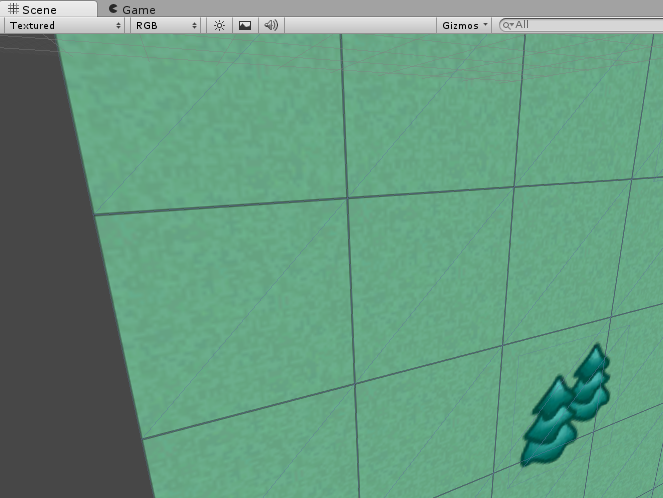# Tiled Map Display Problem

Hi,

I load a tiled map made by Tiled Map Editor, but there are black edges around each tile, how to fix it?The script I used is similar to UniTMX, here is the generating uv part:

``````	private List<Vector2> GenUV (ref TileSet tileset)
{

List<Vector2> uv = new List<Vector2> ();
int horizontalCellCount = (tileset.SourceWidth - tileset.Margin) / (tileset.TileWidth + tileset.Spacing);
int verticalCellCount = (tileset.SourceHeight - tileset.Margin) / (tileset.TileHeight + tileset.Spacing);
float cellWidth = ((float)(tileset.TileWidth) / tileset.SourceWidth);
float cellHeight = ((float)(tileset.TileHeight) / tileset.SourceHeight);
int dataValue;
for (int y = 0; y < Height; y++)
{
for (int x = 0; x < Width; x++)
{
if (Data[y, x] != 0)
{
dataValue = Data[y, x] - tileset.FirstGID;
int posY = dataValue / verticalCellCount;
int posX = dataValue % horizontalCellCount;
float u = ((float)(tileset.Margin + (tileset.TileWidth + tileset.Spacing) * posX) / tileset.SourceWidth);
float v = 1.0f - ((float)(tileset.Margin + (tileset.TileHeight + tileset.Spacing) * posY) / tileset.SourceHeight);
new Vector2 (u, v),
new Vector2 (u, v - cellHeight),
new Vector2 (u + cellWidth, v),
new Vector2 (u + cellWidth, v - cellHeight),
});
}
}
}
return uv;
}
``````

My tileset has 4 pixels for both spacing and margin.